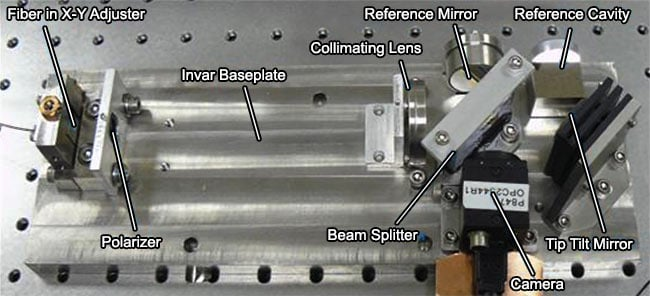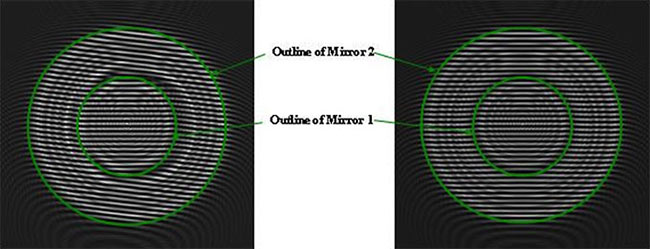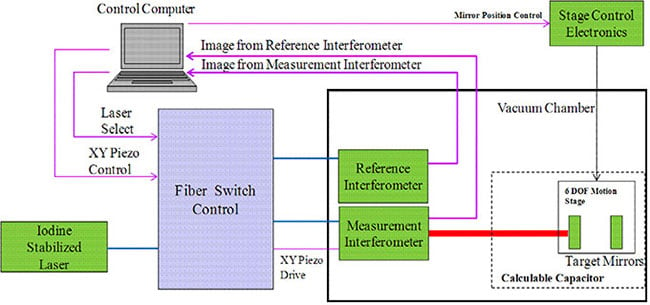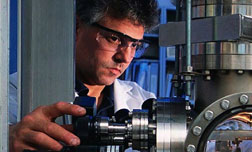Three-Axis Interferometers

Optical Physics Company has worked with the National Institute of Standards and Technology (NIST) on a three-axis interferometer to be used in the NIST calculable capacitor application. The required linear displacement resolution for the three-axis interferometer is 0.1 nm with an angular resolution of 0.1 arc-second.

OPC has assembled the interferometer (shown below).  OPC personnel are currently in the process of finalizing the alignment of this device and characterizing its performance.The basic layout for the 3-axis interferometer is a tip-tilt interferometer shown below.Its operating principles are explained as follows:
The interferometer is configured as a Michelson interferometer where the interference takes place between the return signal from the target mirrors and a fixed reference mirror.

The target mirror’s reflective surfaces are arranged such that one can measure the return from both mirrors simultaneously. A small tilt in the reference mirror produces the interference fringes for each of the target mirrors, and a focal plane array is used to measure the interference patterns.  By measuring the spatial frequency and phase of the interference pattern, one can calculate the change in tip-tilt and piston between the target mirrors and a reference beam splitter.

The expected image on the focal plane is shown below. On the left side of the image the two target mirrors are misaligned and there is a relative tip-tilt between the mirrors. This results in a change of the fringe patterns between the two mirrors which allows for the adjustment of the relative tip-tilt of the two mirrors until they are parallel to each other as shown on the right.Once the tip-tilt angles between the two mirrors are aligned, the relative phase of the two fringe patterns provides a measurement of the distance between the two mirrors. The fringe pattern will change by one cycle when the mirror moves by ½ of laser wavelength.  By measuring the phase of the fringe pattern accurately one can get an accurate measurement of the distance.

An iodine stabilized HeNe laser is used to provide the precision wavelength reference for accurate piston measurements.

Single wavelength measurement of the piston is periodic with a period of λo/2, where λo is the measurement wavelength. In order to resolve this ambiguity, a second measurement wavelength is used. Since the wavelength of the auxiliary laser needs to be known precisely, the assembled instrument includes a reference interferometer to calibrate the auxiliary laser wavelength. A configuration of the final instrument is shown below.Nanometrology ApplicationNational Institute of Standards and Technology (NIST) plays a critical and unique role in the evolution of nanotechnology into nanoscale products and addresses both metrology and manufacturing-related issues.

OPC is working with the NIST Quantum Electrical Metrology Division which maintains the U.S. Farad standard. The Farad standard is based on the NIST calculable capacitor, a unique high-accuracy instrument that directly links the capacitance unit to the mechanical unit of length. The NIST calculable capacitor is the primary standard in the U.S. for the SI measurements of impedance and resistance, and it requires the most precise length measurement techniques.